The variation of the instantaneous current (I) and the instantaneous emf (E) in a circuit is as shown in fig. Which of the following statements is correct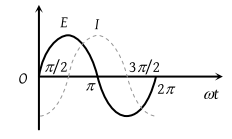(1) The voltage lags behind the current by π / 2

(2) The voltage leads the current by π / 2

(3) The voltage and the current are in phase

(4) The voltage leads the current by π

High Yielding Test Series + Question Bank - NEET 2020

Difficulty Level:

The figure shows the variation of R, XL, and XC with frequency f in a series L, C, R circuit. Then for what frequency point, the circuit is inductive: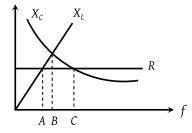(1) A

(2) B

(3) C

(4) All points

Concept Questions :-

Different types of AC Circuits
High Yielding Test Series + Question Bank - NEET 2020

Difficulty Level:

An ac source of variable frequency f is connected to an LCR series circuit. Which one of the graphs in the figure represents the variation of the current I in the circuit with frequency f :

(1)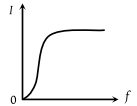(2)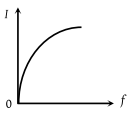(3)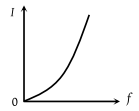(4)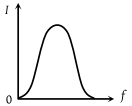Concept Questions :-

Different types of AC Circuits
High Yielding Test Series + Question Bank - NEET 2020

Difficulty Level:

A constant voltage at different frequencies is applied across a capacitance C as shown in the figure. Which of the following graphs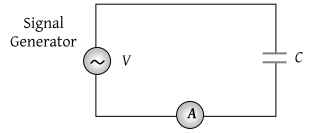Correctly depicts the variation of current with frequency?

(1)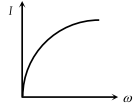(2)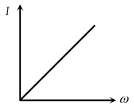(3)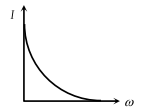(4)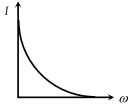High Yielding Test Series + Question Bank - NEET 2020

Difficulty Level:

The output current versus time curve of a rectifier is shown in the figure. The average value of output current in this case is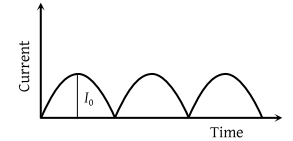(1) 0

(2) $\frac{{I}_{0}}{2}$

(3) $\frac{2{I}_{0}}{\pi }$

(4) I0

High Yielding Test Series + Question Bank - NEET 2020

Difficulty Level:

The current 'i' in an inductance coil varies with time 't' according to the following graph.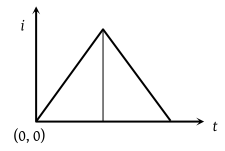Which one of the following plots shows the variations of voltage in the coil?

(1)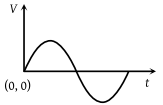(2)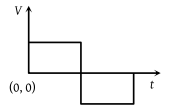(3)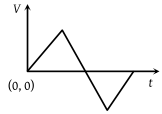(4)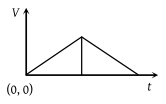Concept Questions :-

Rms and average values
High Yielding Test Series + Question Bank - NEET 2020

Difficulty Level:

When an ac source of e.m.f. $e={E}_{0}\mathrm{sin}\left(100\text{\hspace{0.17em}}t\right)$ is connected across a circuit, the phase difference between the e.m.f. e and the current i in the circuit is observed to be &pi:/4, as shown in the diagram. If the circuit consists possibly only of RC or LC in series, find the relationship between the two elements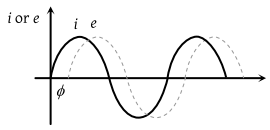(1) $R=1k\Omega ,\text{\hspace{0.17em}}C=10\mu F$

(2) $R=1k\Omega ,\text{\hspace{0.17em}}C=1\mu F$

(3) $R=1k\Omega ,\text{\hspace{0.17em}}L=10H$

(4) $R=1k\Omega ,\text{\hspace{0.17em}}L=1H$

Concept Questions :-

Different types of AC Circuits
High Yielding Test Series + Question Bank - NEET 2020

Difficulty Level:

Two sinusoidal voltages of the same frequency are shown in the diagram. What is the frequency, and the phase relationship between the voltages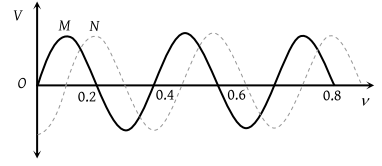Frequency in Hz Phase lead of N over M in radians (1) 0.4 –π/4 (2) 2.5 –π/2 (3) 2.5 +π/2 (4) 2.5 –π/4

Concept Questions :-

AC vs DC
High Yielding Test Series + Question Bank - NEET 2020

Difficulty Level:

Which of the following plots may represent the reactance of a series LC combination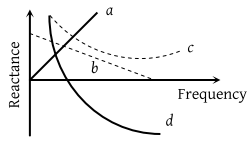(1) a

(2) b

(3) c

(4) d

Concept Questions :-

Different types of AC Circuits
High Yielding Test Series + Question Bank - NEET 2020

Difficulty Level:

A filament bulb (500 W,100 V) is to be used in a 230 V main supply. When a resistance R is connected in series, it works perfectly and the bulb consumes 500 W. The value of R is

(a) 230$\Omega$          (b) 46$\Omega$

(c) 26$\Omega$            (d) 13$\Omega$